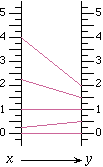# Function Diagram WorksheetsHenri Picciotto

For an introduction to function diagrams, some teacher notes,
and a context for these worksheets, see Function Diagrams.

Basics:
The table, graph, and function diagram for y = 2x - 3
Introduction to functions: Guess My Function
Blank tables and function diagrams
From Algebra: Themes, Tools, Concepts
Operations, signed numbers, and linear functions: Nine Function Diagrams
y = mx + b: Focus on Function Diagrams, and Sixteen Function Diagrams
Cartesian graph / function diagram duality: Representing Linear Functions
Full list of ATTC lessons: 2.7, 2.8, 2.9, 3.2, 3.10, 3.B, 3.C, 5.1, 5.7, 8.2, 9.5, 12.7, 12.8
An application of function diagrams:
Iterating Linear Functions
Domain, Range, Magnification, and Composition:
Six Function Diagrams — Algebra 1 or Algebra 2
Eight Function Diagrams — end of Algebra 2, Precalculus, or beginning of Calculus
Composition and magnification — the topics are suitable for Algebra 2 and beyond, but the worksheet is best for teachers
The focus (proof — mostly for teachers)
Geometry of Function DiagramsRelated pages on this site:
Function diagrams overview
Function diagram applets
Electronic tools for function diagrams